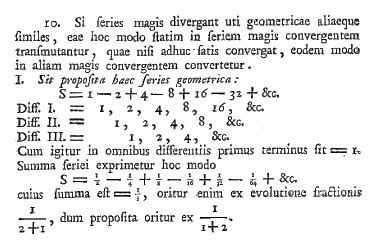# 1 − 2 4 − 8 ⋯

Updated onIn mathematics, 1 − 2 + 4 − 8 + ⋯ is the infinite series whose terms are the successive powers of two with alternating signs. As a geometric series, it is characterized by its first term, 1, and its common ratio, −2.

## Contents

k = 0 n ( 2 ) k

As a series of real numbers it diverges, so in the usual sense it has no sum. In a much broader sense, the series has a generalized sum of 1/3.

## Historical arguments

Gottfried Leibniz considered the divergent alternating series 1 − 2 + 4 − 8 + 16 − ⋯ as early as 1673. He argued that by subtracting either on the left or on the right, one could produce either positive or negative infinity, and therefore both answers are wrong and the whole should be finite:

Now normally nature chooses the middle if neither of the two is permitted, or rather if it cannot be determined which of the two is permitted, and the whole is equal to a finite quantity

Leibniz did not quite assert that the series had a sum, but he did infer an association with 1/3 following Mercator's method. The attitude that a series could equal some finite quantity without actually adding up to it as a sum would be commonplace in the 18th century, although no distinction is made in modern mathematics.

After Christian Wolff read Leibniz's treatment of Grandi's series in mid-1712, Wolff was so pleased with the solution that he sought to extend the arithmetic mean method to more divergent series such as 1 − 2 + 4 − 8 + 16 − ⋯. Briefly, if one expresses a partial sum of this series as a function of the penultimate term, one obtains either 4m + 1/3 or −4n + 1/3. The mean of these values is 2m − 2n + 1/3, and assuming that m = n at infinity yields 1/3 as the value of the series. Leibniz's intuition prevented him from straining his solution this far, and he wrote back that Wolff's idea was interesting but invalid for several reasons. The arithmetic means of neighboring partial sums do not converge to any particular value, and for all finite cases one has n = 2m, not n = m. Generally, the terms of a summable series should decrease to zero; even 1 − 1 + 1 − 1 + ⋯ could be expressed as a limit of such series. Leibniz counsels Wolff to reconsider so that he "might produce something worthy of science and himself."

## Geometric series

Any summation method possessing the properties of regularity, linearity, and stability will sum a geometric series

k = 0 a r k = a 1 r .

In this case a = 1 and r = −2, so the sum is 1/3.

## Euler summation

In his 1755 Institutiones, Leonhard Euler effectively took what is now called the Euler transform of 1 − 2 + 4 − 8 + ⋯, arriving at the convergent series 1/21/4 + 1/81/16 + ⋯. Since the latter sums to 1/3, Euler concluded that 1 − 2 + 4 − 8 + ... = 1/3. His ideas on infinite series do not quite follow the modern approach; today one says that 1 − 2 + 4 − 8 + ... is Euler summable and that its Euler sum is 1/3.

The Euler transform begins with the sequence of positive terms:

a0 = 1, a1 = 2, a2 = 4, a3 = 8,...

The sequence of forward differences is then

Δa0 = a1a0 = 2 − 1 = 1, Δa1 = a2a1 = 4 − 2 = 2, Δa2 = a3a2 = 8 − 4 = 4, Δa3 = a4a3 = 16 − 8 = 8,...

which is just the same sequence. Hence the iterated forward difference sequences all start with Δna0 = 1 for every n. The Euler transform is the series

a 0 2 Δ a 0 4 + Δ 2 a 0 8 Δ 3 a 0 16 + = 1 2 1 4 + 1 8 1 16 + .

This is a convergent geometric series whose sum is 1/3 by the usual formula.

## Borel summation

The Borel sum of 1 − 2 + 4 − 8 + ⋯ is also 1/3; when Émile Borel introduced the limit formulation of Borel summation in 1896, this was one of his first examples after 1 − 1 + 1 − 1 + ⋯

1 − 2 + 4 − 8 + ⋯ Wikipedia